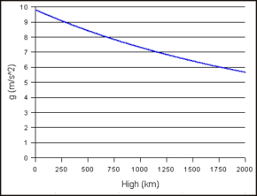## How to Calculate and Solve for Gravitational Acceleration | GravityThe image above represents gravitational acceleration.

To compute for gravitational acceleration, three essential parameters are needed and these parameters are Gravitational Constant (G), Mass of the Earth (mEand Radius of the Earth (R).

The formula for calculating gravitational acceleration:

a = GmE /

Where:

a = Gravitational Acceleration
G = Gravitational Constant
mE = Mass of the Earth
R = Radius of the Earth

Let’s solve an example;
Find the Gravitational acceleration when the gravitational constant is 6.67E-11, the mass of the earth is 12 and the radius of the earth is 21.

This implies that;

G = Gravitational Constant = 6.67E-11
mE = Mass of the Earth = 12
R = Radius of the Earth = 21

a = GmE /
a = (6.67e-11)(12) / (21)²
a = 8.004e-10 / 441
a = 1.81e-12

Therefore, the gravitational acceleration is 1.81e-12 m/s.